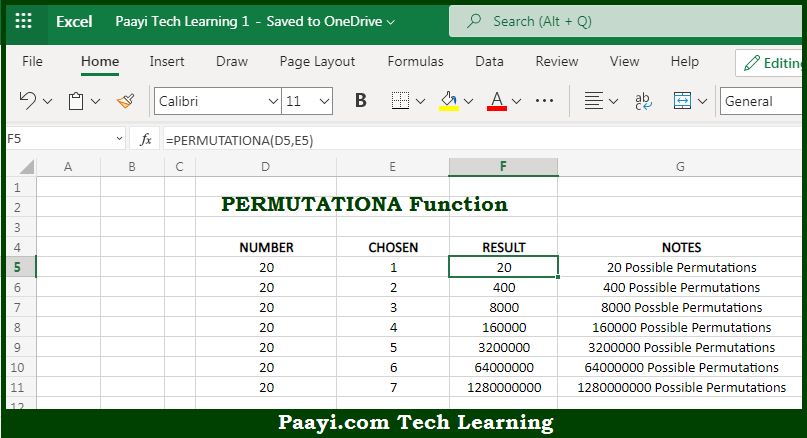# Learn How to Use Microsoft Excel PERMUTATIONA Function

Written by | 0 Comments | 642 Views

In this article, you will learn how to use the Microsoft Excel PERMUTATIONA function and its prime function in Microsoft Excel. You will also get to know the Microsoft Excel PERMUTATIONA function return value and syntax with the help of some examples.

Microsoft Excel PERMUTATIONA Function

The main purpose of the Microsoft Excel PERMUTATIONA function is to get the number of permutations with repetitions. That implies, with the help of PERMUTATIONA function you can able to return the number of permutations, which are the combinations where the order is significant for a given number of items. It should be noted that PERMUTATIONA function allows repetitions. In case you want to allow permutations without repetitions, use the PERMUT function. So, with the help of PERMUTATIONA function, you can able to get the number of permutations with repetitions.

Return Value of PERMUTATIONA Function

The return value will be the number of permutations with repetitions.

Syntax of PERMUTATIONA Function

=PERMUTATIONA(number, number-chosen)

Where the arguments:

• number: This is the total number of items.
• number-chosen: This is the number of items in each combination.

How to Use Microsoft Excel PERMUTATIONA Function?So we know that Microsoft Excel PERMUTATIONA function you can able to get the number of permutations with repetitions. That implies, with the help of PERMUTATIONA function you can able to return the number of permutations, which are the combinations where an order is significant for a given number of items. It should be noted that PERMUTATIONA function allows repetitions. In case you want to allow permutations without repetitions, use the PERMUT function. So, with the help of PERMUTATIONA function, you can able to get the number of permutations with repetitions.